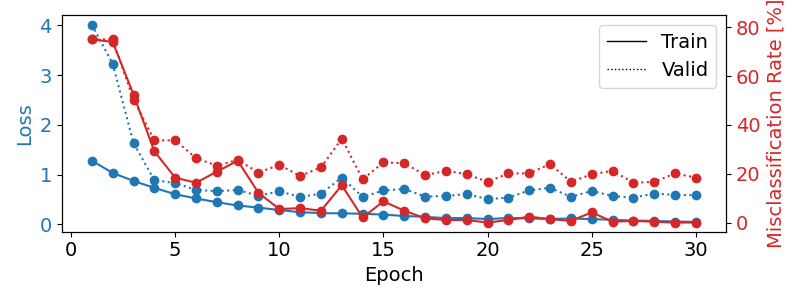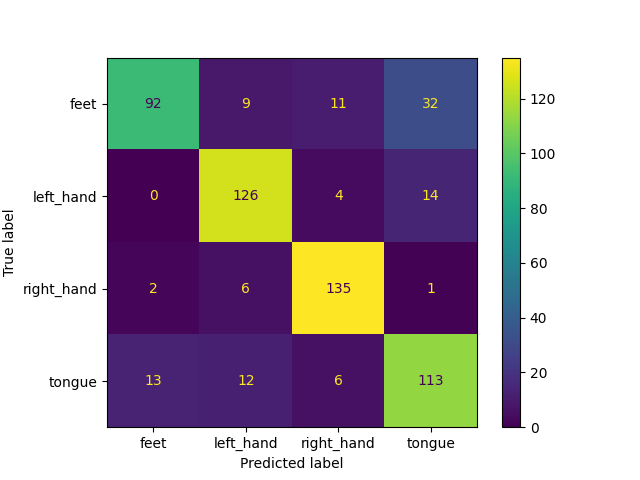# Cropped Decoding on BCIC IV 2a Dataset#

Building on the Trialwise decoding tutorial, we now do more data-efficient cropped decoding!

```# In Braindecode, there are two supported configurations created for
# training models: trialwise decoding and cropped decoding. We will
# explain this visually by comparing trialwise to cropped decoding.
#
# .. image:: ../_static/trialwise_explanation.png
# .. image:: ../_static/cropped_explanation.png
#
# On the left, you see trialwise decoding:
#
# 1. A complete trial is pushed through the network.
# 2. The network produces a prediction.
# 3. The prediction is compared to the target (label) for that trial to
#    compute the loss.
#
# On the right, you see cropped decoding:
#
# 1. Instead of a complete trial, crops are pushed through the network.
# 2. For computational efficiency, multiple neighbouring crops are pushed
#    through the network simultaneously (these neighbouring crops are
#    called compute windows)
# 3. Therefore, the network produces multiple predictions (one per crop in
#    the window)
# 4. The individual crop predictions are averaged before computing the
#    loss function
#
# .. note::
#
#     -  The network architecture implicitly defines the crop size (it is the
#        receptive field size, i.e., the number of timesteps the network uses
#        to make a single prediction)
#     -  The window size is a user-defined hyperparameter, called
#        ``input_window_samples`` in Braindecode. It mostly affects runtime
#        (larger window sizes should be faster). As a rule of thumb, you can
#        set it to two times the crop size.
#     -  Crop size and window size together define how many predictions the
#        network makes per window: ``#window − #crop + 1 = #predictions``
#
```
```# .. note::
#
#     For cropped decoding, the above training setup is mathematically
#     identical to sampling crops in your dataset, pushing them through the
#     network and training directly on the individual crops. At the same time,
#     the above training setup is much faster as it avoids redundant
#     computations by using dilated convolutions, see our paper
#     `Deep learning with convolutional neural networks for EEG decoding and visualization <https://arxiv.org/abs/1703.05051>`_. # noqa: E501
#     However, the two setups are only mathematically identical in case (1)
#     to the same gradients when using the averaged output. The first is true
#     for our shallow and deep ConvNet models and the second is true for the
#     log-softmax outputs and negative log likelihood loss that is typically
#     used for classification in PyTorch.
#

# -------------------------------------
#
# Loading and preprocessing stays the same as in the `Trialwise decoding
# tutorial <./plot_bcic_iv_2a_moabb_trial.html>`__.

from braindecode.datasets import MOABBDataset

subject_id = 3
dataset = MOABBDataset(dataset_name="BNCI2014001", subject_ids=[subject_id])

from braindecode.preprocessing import (
exponential_moving_standardize, preprocess, Preprocessor)
from numpy import multiply

low_cut_hz = 4.  # low cut frequency for filtering
high_cut_hz = 38.  # high cut frequency for filtering
# Parameters for exponential moving standardization
factor_new = 1e-3
init_block_size = 1000
# Factor to convert from V to uV
factor = 1e6

preprocessors = [
Preprocessor('pick_types', eeg=True, meg=False, stim=False),  # Keep EEG sensors
Preprocessor(lambda data: multiply(data, factor)),  # Convert from V to uV
Preprocessor('filter', l_freq=low_cut_hz, h_freq=high_cut_hz),  # Bandpass filter
Preprocessor(exponential_moving_standardize,  # Exponential moving standardization
factor_new=factor_new, init_block_size=init_block_size)
]

# Transform the data
preprocess(dataset, preprocessors)
```
```/home/runner/work/braindecode/braindecode/braindecode/preprocessing/preprocess.py:55: UserWarning: Preprocessing choices with lambda functions cannot be saved.
warn('Preprocessing choices with lambda functions cannot be saved.')

<braindecode.datasets.moabb.MOABBDataset object at 0x7f50a90f6350>
```

## Create model and compute windowing parameters#

In contrast to trialwise decoding, we first have to create the model before we can cut the dataset into windows. This is because we need to know the receptive field of the network to know how large the window stride should be.

```# We first choose the compute/input window size that will be fed to the
# network during training This has to be larger than the networks
# receptive field size and can otherwise be chosen for computational
# efficiency (see explanations in the beginning of this tutorial). Here we
# choose 1000 samples, which are 4 seconds for the 250 Hz sampling rate.
#

input_window_samples = 1000
```

Now we create the model. To enable it to be used in cropped decoding efficiently, we manually set the length of the final convolution layer to some length that makes the receptive field of the ConvNet smaller than `input_window_samples` (see `final_conv_length=30` in the model definition).

```import torch
from braindecode.util import set_random_seeds
from braindecode.models import ShallowFBCSPNet

cuda = torch.cuda.is_available()  # check if GPU is available, if True chooses to use it
device = 'cuda' if cuda else 'cpu'
if cuda:
torch.backends.cudnn.benchmark = True
# Set random seed to be able to roughly reproduce results
# Note that with cudnn benchmark set to True, GPU indeterminism
# may still make results substantially different between runs.
# To obtain more consistent results at the cost of increased computation time,
# you can set `cudnn_benchmark=False` in `set_random_seeds`
# or remove `torch.backends.cudnn.benchmark = True`
seed = 20200220
set_random_seeds(seed=seed, cuda=cuda)

n_classes = 4
# Extract number of chans from dataset
n_chans = dataset.shape

model = ShallowFBCSPNet(
n_chans,
n_classes,
input_window_samples=input_window_samples,
final_conv_length=30,
)

# Send model to GPU
if cuda:
model.cuda()
```

And now we transform model with strides to a model that outputs dense prediction, so we can use it to obtain predictions for all crops.

```from braindecode.models import to_dense_prediction_model, get_output_shape

to_dense_prediction_model(model)
```

To know the models’ receptive field, we calculate the shape of model output for a dummy input.

```n_preds_per_input = get_output_shape(model, n_chans, input_window_samples)
```

## Cut the data into windows#

In contrast to trialwise decoding, we have to supply an explicit window size and window stride to the `create_windows_from_events` function.

```from braindecode.preprocessing import create_windows_from_events

trial_start_offset_seconds = -0.5
# Extract sampling frequency, check that they are same in all datasets
sfreq = dataset.datasets.raw.info['sfreq']
assert all([ds.raw.info['sfreq'] == sfreq for ds in dataset.datasets])

# Calculate the trial start offset in samples.
trial_start_offset_samples = int(trial_start_offset_seconds * sfreq)

# Create windows using braindecode function for this. It needs parameters to define how
# trials should be used.
windows_dataset = create_windows_from_events(
dataset,
trial_start_offset_samples=trial_start_offset_samples,
trial_stop_offset_samples=0,
window_size_samples=input_window_samples,
window_stride_samples=n_preds_per_input,
drop_last_window=False,
)
```

## Split the dataset#

This code is the same as in trialwise decoding.

```splitted = windows_dataset.split('session')
train_set = splitted['session_T']
valid_set = splitted['session_E']
```
Training

In difference to trialwise decoding, we now should supply `cropped=True` to the EEGClassifier, and `CroppedLoss` as the criterion, as well as `criterion__loss_function` as the loss function applied to the meaned predictions.

```# .. note::
#
#     In this tutorial, we use some default parameters that we
#     have found to work well for motor decoding, however we strongly
#     encourage you to perform your own hyperparameter optimization using
#     cross validation on your training data.
#

from skorch.callbacks import LRScheduler
from skorch.helper import predefined_split

from braindecode import EEGClassifier
from braindecode.training import CroppedLoss

# These values we found good for shallow network:
lr = 0.0625 * 0.01
weight_decay = 0

# For deep4 they should be:
# lr = 1 * 0.01
# weight_decay = 0.5 * 0.001

batch_size = 64
n_epochs = 4

clf = EEGClassifier(
model,
cropped=True,
criterion=CroppedLoss,
criterion__loss_function=torch.nn.functional.nll_loss,
train_split=predefined_split(valid_set),
optimizer__lr=lr,
optimizer__weight_decay=weight_decay,
iterator_train__shuffle=True,
batch_size=batch_size,
callbacks=[
"accuracy", ("lr_scheduler", LRScheduler('CosineAnnealingLR', T_max=n_epochs - 1)),
],
device=device,
)
# Model training for a specified number of epochs. `y` is None as it is already supplied
# in the dataset.
clf.fit(train_set, y=None, epochs=n_epochs)
```
```/usr/share/miniconda/envs/braindecode/lib/python3.7/site-packages/skorch/callbacks/base.py:146: SkorchWarning: You are using an callback that overrides on_batch_begin or on_batch_end. As of skorch 0.10, the signature was changed from 'on_batch_{begin,end}(self, X, y, ...)' to 'on_batch_{begin,end}(self, batch, ...)'. To recover, change the signature accordingly and add 'X, y = batch' on the first line of the method body. To suppress this warning, add:
'import warnings; from skorch.exceptions import SkorchWarning
warnings.filterwarnings('ignore', category=SkorchWarning)'.
warnings.warn(warning_msg, SkorchWarning)
epoch    train_accuracy    train_loss    valid_accuracy    valid_loss      lr      dur
-------  ----------------  ------------  ----------------  ------------  ------  -------
1            0.2500        1.4486            0.2500        5.4385  0.0006  17.6361
2            0.2569        1.2315            0.2500        3.6823  0.0005  17.2271
3            0.3958        1.1476            0.3611        2.2702  0.0002  17.1282
4            0.4340        1.1225            0.4410        1.4375  0.0000  17.1336

<class 'braindecode.classifier.EEGClassifier'>[initialized](
module_=ShallowFBCSPNet(
(ensuredims): Ensure4d()
(dimshuffle): Expression(expression=transpose_time_to_spat)
(conv_time): Conv2d(1, 40, kernel_size=(25, 1), stride=(1, 1))
(conv_spat): Conv2d(40, 40, kernel_size=(1, 22), stride=(1, 1), bias=False)
(bnorm): BatchNorm2d(40, eps=1e-05, momentum=0.1, affine=True, track_running_stats=True)
(conv_nonlin_exp): Expression(expression=square)
(pool): AvgPool2d(kernel_size=(75, 1), stride=(1, 1), padding=0)
(pool_nonlin_exp): Expression(expression=safe_log)
(drop): Dropout(p=0.5, inplace=False)
(conv_classifier): Conv2d(40, 4, kernel_size=(30, 1), stride=(1, 1), dilation=(15, 1))
(softmax): LogSoftmax(dim=1)
(squeeze): Expression(expression=squeeze_final_output)
),
)
```

## Plot Results#

### This is again the same code as in trialwise decoding.#

Note

Note that we drop further in the classification error and loss as in the trialwise decoding tutorial.

```import matplotlib.pyplot as plt
from matplotlib.lines import Line2D
import pandas as pd

# Extract loss and accuracy values for plotting from history object
results_columns = ['train_loss', 'valid_loss', 'train_accuracy', 'valid_accuracy']
df = pd.DataFrame(clf.history[:, results_columns], columns=results_columns,
index=clf.history[:, 'epoch'])

# get percent of misclass for better visual comparison to loss
df = df.assign(train_misclass=100 - 100 * df.train_accuracy,
valid_misclass=100 - 100 * df.valid_accuracy)

plt.style.use('seaborn')
fig, ax1 = plt.subplots(figsize=(8, 3))
df.loc[:, ['train_loss', 'valid_loss']].plot(
ax=ax1, style=['-', ':'], marker='o', color='tab:blue', legend=False, fontsize=14)

ax1.tick_params(axis='y', labelcolor='tab:blue', labelsize=14)
ax1.set_ylabel("Loss", color='tab:blue', fontsize=14)

ax2 = ax1.twinx()  # instantiate a second axes that shares the same x-axis

df.loc[:, ['train_misclass', 'valid_misclass']].plot(
ax=ax2, style=['-', ':'], marker='o', color='tab:red', legend=False)
ax2.tick_params(axis='y', labelcolor='tab:red', labelsize=14)
ax2.set_ylabel("Misclassification Rate [%]", color='tab:red', fontsize=14)
ax2.set_ylim(ax2.get_ylim(), 85)  # make some room for legend
ax1.set_xlabel("Epoch", fontsize=14)

# where some data has already been plotted to ax
handles = []
handles.append(Line2D(, , color='black', linewidth=1, linestyle='-', label='Train'))
handles.append(Line2D(, , color='black', linewidth=1, linestyle=':', label='Valid'))
plt.legend(handles, [h.get_label() for h in handles], fontsize=14)
plt.tight_layout()
```## Plot Confusion Matrix#

Generate a confusion matrix as in https://onlinelibrary.wiley.com/doi/full/10.1002/hbm.23730

```from sklearn.metrics import confusion_matrix
from braindecode.visualization import plot_confusion_matrix

# generate confusion matrices
# get the targets
y_pred = clf.predict(valid_set)

# generating confusion matrix
confusion_mat = confusion_matrix(y_true, y_pred)

# label_dict is class_name : str -> i_class : int
label_dict = valid_set.datasets.windows.event_id.items()
# sort the labels by values (values are integer class labels)
labels = list(dict(sorted(list(label_dict), key=lambda kv: kv)).keys())

# plot the basic conf. matrix
plot_confusion_matrix(confusion_mat, class_names=labels)
``````<Figure size 800x550 with 1 Axes>
```

Total running time of the script: ( 1 minutes 41.173 seconds)

Estimated memory usage: 864 MB

Gallery generated by Sphinx-Gallery